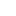# Unit 5: Cost Functions

## Introduction

What’s in This Chapter? One of a firm’s objectives is to be cost efficient and thus increasing a firm’s profits. This unit analyzes a firm’s costs of production. A firm’s fixed costs include its...

## Section 1: Explicit Costs and Implicit Costs

The Difference Between Explicit and Implicit Costs Economists distinguish between explicit and implicit costs. Explicit costs, also called accounting costs, are out-of-pocket costs, such as expenses on labor, raw materials, and...

## Section 2: Accounting versus Economic Profits

Economic Costs and Accounting Costs Below are definitions of economic costs and accounting costs. Economic costs = implicit costs + explicit costs Accounting costs = explicit costs Economic Profit and Accounting Profit Below are...

## Section 3: Total and Per Unit Costs

Economic Costs There are seven important economic costs: Total Variable Cost (TVC). This is the cost of all variable inputs. Examples include the cost of temporary workers and hourly labor, the cost of materials, office...

## Section 4: Cost Calculations

What are the Relationships Between the Various Costs? Section 3 provides definitions of the important economic costs. Below is a list of the relationships between these costs. Using the abbreviations from the previous section,...

## Section 5: Cost Curves

Graphing Cost Data from a Table In the previous section, we learned how to calculate cost data. Below we will plot the data in a graph. The next diagram shows a hypothetical firm’s total cost, total variable cost, and...

## Section 6: The Long-Run Average Cost Curve

Derivation of the Long-Run Average Cost Curve A firm’s long-run average cost curve is derived from its short-run average cost curves. For each short-run fixed plant size, we take the lowest or near-lowest costs for that...

## Section 7: Increasing, Decreasing, and Constant Returns to Scale

Increasing Returns to Scale Increasing returns to scale is closely associated with economies of scale (the downward sloping part of the long-run average total cost curve in the previous section). Increasing returns to scale...